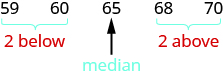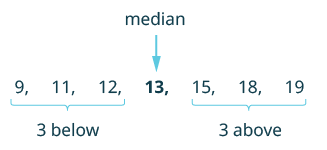## Finding the Median of a Set of Numbers

### Learning Outcomes

• Find the median of a set of numbers

When Ann, Bianca, Dora, Eve, and Francine sing together on stage, they line up in order of their heights. Their heights, in inches, are shown in the table below.

Ann Bianca Dora Eve Francine
$59$ $60$ $65$ $68$ $70$

Dora is in the middle of the group. Her height, $65″$, is the median of the girls’ heights. Half of the heights are less than or equal to Dora’s height, and half are greater than or equal. The median is the middle value.### Median

The median of a set of data values is the middle value.

• Half the data values are less than or equal to the median.
• Half the data values are greater than or equal to the median.

What if Carmen, the pianist, joins the singing group on stage? Carmen is $62$ inches tall, so she fits in the height order between Bianca and Dora. Now the data set looks like this:

$59,60,62,65,68,70$

There is no single middle value. The heights of the six girls can be divided into two equal parts.Statisticians have agreed that in cases like this the median is the mean of the two values closest to the middle. So the median is the mean of $62\text{ and }65,{\Large\frac{62+65}{2}}$. The median height is $63.5$ inches.Notice that when the number of girls was $5$, the median was the third height, but when the number of girls was $6$, the median was the mean of the third and fourth heights. In general, when the number of values is odd, the median will be the one value in the middle, but when the number is even, the median is the mean of the two middle values.

### Find the median of a set of numbers.

1. List the numbers from smallest to largest.
2. Count how many numbers are in the set. Call this $n$.
3. Is $n$ odd or even?
• If $n$ is an odd number, the median is the middle value.
• If $n$ is an even number, the median is the mean of the two middle values.

### example

Find the median of $12,13,19,9,11,15,\text{and }18$.

Solution

 List the numbers in order from smallest to largest. $9, 11, 12, 13, 15, 18, 19$ Count how many numbers are in the set. Call this $n$ . $n=7$ Is $n$ odd or even? odd The median is the middle value.The middle is the number in the $4$th position. So the median of the data is $13$.

### example

Kristen received the following scores on her weekly math quizzes:
$83,79,85,86,92,100,76,90,88,\text{and }64$. Find her median score.

### try it

The next video shows another example of how to find hte median of a set of numbers.

## Contribute!

Did you have an idea for improving this content? We’d love your input.